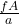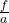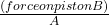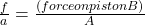A piston of small cross-sectional area a is used in a hydraulic press to exert a small force f on the enclosed liquid. A connecting pipe lea

Question

A piston of small cross-sectional area a is used in a hydraulic press to exert a small force f on the enclosed liquid. A connecting pipe leads to a larger piston of cross sectional area A. a) What force F will the larger piston sustain

in progress 0
5 months 2021-08-22T11:59:27+00:00 1 Answers 5 views 0

force on larger piston =Explanation:

we label the pistons as piston A and piston B

small piston A:

area = a

force = f

large piston B:

area = A

force  = ?

Pascal’s law of pressure state that the pressure delivered to a liquid is transmitted undiminished to every portion of the fluid.

we know that pressure = force ÷ area

pressure of piston A =pressure of piston B =obeying Pascal’s law, the system pressures must be equal. Thereforeforce on large piston (B) = F =